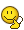# Multiplication of Taylor and Laurent series

• LagrangeEuler
In summary, the first series is\frac{1}{2}\sum^{\infty}_{n=0}\frac{(-1)^n}{n+1}(\frac{1}{p^2})^{n+1}= \frac{1}{2}(\frac{1}{p^2}-\frac{1}{2p^4}+\frac{1}{3p^6}-\frac{1}{4p^8}+...)while the second series is\sum^{\infty}_{k=0}\frac{p^kt^k}{k!}=1+pt+\frac{p^2t^2f

#### LagrangeEuler

Homework Statement
Find coefficient which multiply ##\frac{1}{p}## in product of series. Coefficient is function of ##t##.
$$\frac{1}{2}\sum^{\infty}_{n=0}\frac{(-1)^n}{n+1}(\frac{1}{p^2})^{n+1}\,\sum^{\infty}_{k=0}\frac{p^kt^k}{k!}$$
Namely product of two series will be new series that will contain the sum of terms of ##p^l## where ##l## are integers. I want to find only the term ##f(t)\frac{1}{p}## in the product.
Relevant Equations
I think that the sum of terms that multiply ##1/p## in product of two series should be ##\frac{1-\cos t}{t}##.
First series
$$\frac{1}{2}\sum^{\infty}_{n=0}\frac{(-1)^n}{n+1}(\frac{1}{p^2})^{n+1}= \frac{1}{2}(\frac{1}{p^2}-\frac{1}{2p^4}+\frac{1}{3p^6}-\frac{1}{4p^8}+...)$$
whereas second one is
$$\sum^{\infty}_{k=0}\frac{p^kt^k}{k!}=1+pt+\frac{p^2t^2}{2!}+\frac{p^3t^3}{3!}+\frac{p^4t^4}{4!}+\frac{p^5t^5}{5!}+...$$.
I multiply two series and I want to get coefficient that multiply ##1/p## in the series that I get as a product. I will get this type of term if I multiply ##\frac{1}{p^2}## with ##p##, ##\frac{1}{p^4}## with ##p^3##... And I need to sum all these terms.
I want to see what is coefficient that multiplies ##\frac{1}{p}## in the expansion of product of two series. That coefficient is function of ##t##.
First term I get by multiplying ##\frac{1}{2p^2}## and ##pt## and it is ##\frac{t}{2}##.
Second term I get by multiplying ##-\frac{1}{4p^4}## and ##\frac{p^3t^3}{3!}## and it is ##-\frac{t^3}{4!}##
Third term will be ##\frac{t^5}{6!}##...
I think that the sum should be ##\frac{1-\cos t}{t}##. Is there some easy way to get it without writing down all the sums?

Last edited:
Please render the complete problem statement. I now see four variables ( ##k, n, p, t ## ) and there are two summation indices ( ##n, k ## ). How can the result not depend on ##p## ?

And what do you mean with
I want to see what stands in front of ##1/p##

And (hint): do you know the series for ##e^x## ?

##\ ##

I write down two series. Yes, I know. But I shouldn't go back. If I multiply two series and then asked
what is a coefficient that multiplies ##1/p## in the product of two series?
It is ##f(t)\frac{1}{p}## and ##f(t)=\frac{1-\cos t}{t}##. But I have problem to derive ##f(t)## from series multiplication. I rewrite problem statement a bit. I hope that now is more clear. ##n,k## are just sumational dummy indices.

I hope that now is more clear.
Not to me.
Please render the complete problem statement.
As it was given to you. Verbatim

##\ ##

Not to me.

As it was given to you. Verbatim

Please render the complete problem statement. I now see four variables ( ##k, n, p, t ## ) and there are two summation indices ( ##n, k ## ). How can the result not depend on ##p## ?
The problem is only asking for the coefficient of the 1/p term of the Laurent series, which is not a function of p.
I think that the sum should be ##\frac{1-\cos t}{t}##. Is there some easy way to get it without writing down all the sums?
Not in general. I have not checked the details of your work, but I think it is the approach they had in mind.

•BvU and LagrangeEuler
I tried to edit it once again. I multiply two series. I will get some third series which will be
$$f(t)\frac{1}{p}+...$$
I gave in the statement both series that I multiply in order to get the third one. And I need to find ##f(t)##.

Why don' t you pursuit your way to get the coefficient of 1/p,
$$\frac{1}{2}\sum_{n=1}^\infty \frac{t^{2n-1}}{(2n-1)!}\frac{(-1)^{n+1}}{n}$$
and take a look at it?

You see terms n=1 and n=2 are the same as you have got.

Last edited:
•LagrangeEuler
Ah, I finally understand the problem statementSorry it took me a while, and I second @anuttarasammyak . Whether that qualifies as 'some easy way to get it without writing down all the sums' is subjective##\ ##

•LagrangeEuler
I can do this in that fashion, however, I would like to try to get this sum immediately without using induction. Can you show me how to obtain this by playing with the sums?

From the formula of #8
$$\frac{1}{t}[1-\sum_{n=0}^\infty \frac{(-1)^nt^{2n}}{(2n)!}]$$

Last edited:
I can not get this sum from the product. Can you please show me how to get this? Without multiplying term by term.

Just do what you described in your original post. For each value of ##n##, pick off just the term in the second series so the product is a ##p^{-1}## term. The first series contributes ##p^{-(2n+2)}##, so you want the term where ##k=2n+1## from the second series.